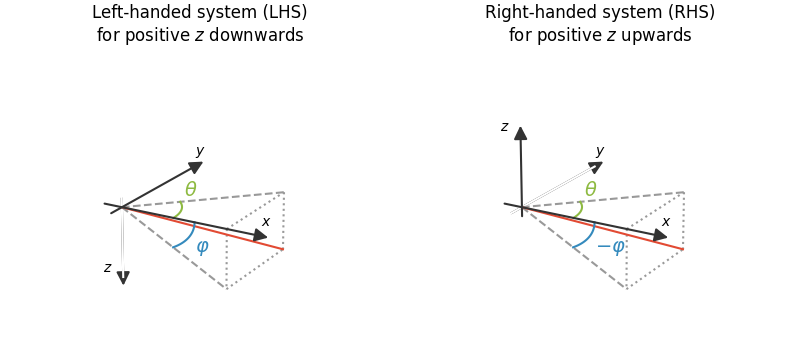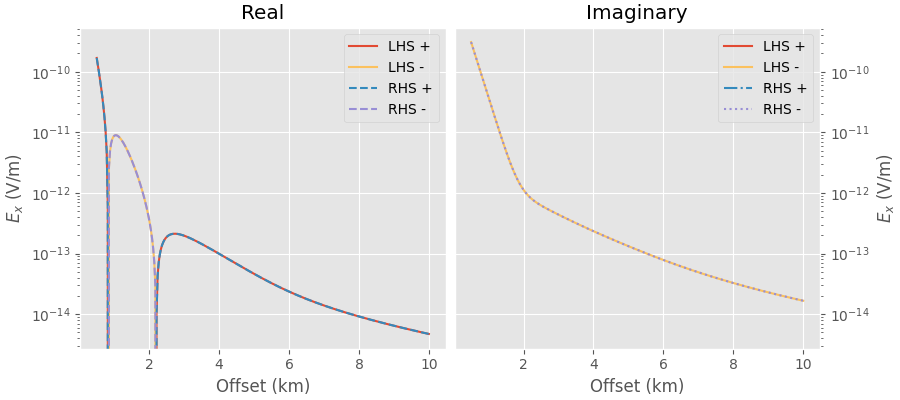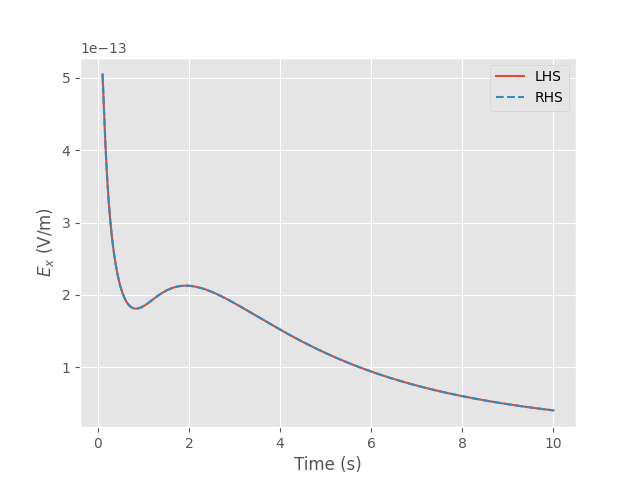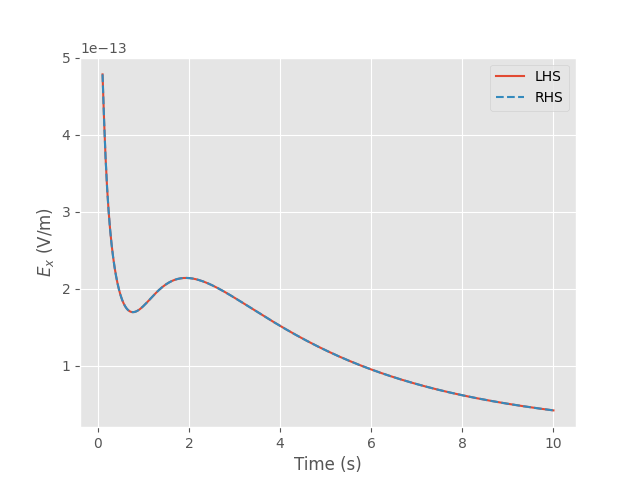# Definition of the coordinate system in empymod#

## Short version#

The used coordinate system is either a

• Left-Handed System (LHS), where Easting is the $$x$$-direction, Northing the $$y$$-direction, and positive $$z$$ is pointing downwards;

• Right-Handed System (RHS), where Easting is the $$x$$-direction, Northing the $$y$$-direction, and positive $$z$$ is pointing upwards.

## In more detail#

The derivation on which empymod is based ([HuTS15]) uses a right-handed system with $$x$$ to the East, $$y$$ to the South, and $$z$$ downwards (ESD). In the actual original implementation of empymod this was changed to a left-handed system with $$x$$ to the East, $$y$$ to the North, and $$z$$ downwards (END). However, empymod can equally well be used for a coordinate system where positive $$z$$ is pointing up by just flipping $$z$$, resulting in $$x$$ to the East, $$y$$ to the North, and $$z$$ upwards (ENU).

Left-handed system

Right-handed system

$$x$$

Easting

Easting

$$y$$

Northing

Northing

$$z$$

Down

Up

$$\theta$$

Angle E-N

Angle E-N

$$\varphi$$

Angle down

Angle up

There are a few other important points to keep in mind when switching between coordinate systems:

• The interfaces (depth) have to be defined continuously increasing or decreasing. E.g., think of a simple five-layer model with the sea-surface at 0 m, a 100 m water column, and a target of 50 m 900 m below the seafloor, with the following resistivities:

• res = [1e12, 0.3, 1, 50, 1]: air, water, background, target, background.

We can now define the depths in two different ways:

• depth = [0, 100, 1000, 1050]: LHS (+z down)

• depth = [0, -100, -1000, -1050]: RHS (+z up)

• A source or a receiver exactly on a boundary is taken as being in the lower layer. Hence, if $$z_\rm{rec} = z_0$$, where $$z_0$$ is the surface, then the receiver is taken as in the air in the LHS, but as in the subsurface in the RHS. Similarly, if $$z_\rm{rec} = z_\rm{seafloor}$$, then the receiver is taken as in the sea in the LHS, but as in the subsurface in the RHS. This can be avoided by never placing it exactly on a boundary, but slightly (e.g., 1 mm) in the layer where you want to have it.

• Sign switches:

• In bipole, the dip switches sign.

• In dipole, the ab’s containing vertical directions switch the sign for each vertical component.

• Sign switches also occur for magnetic sources or receivers.

These sign switches multiply; e.g., ab=34 has no sign switch, as the switch due to the vertical source and the switch due to the magnetic receiver cancel each other. Here an overview of the sign switches (–) for dipole when changing from the default LHS to a RHS:

electric source

magnetic source

x

y

z

x

y

z

electric

x

y

z

magnetic

x

y

z

• The depths can also be defined the other way around, starting at +/-1050 going to 0. You have to change the order of all model parameters (res, aniso, {e;m}perm{H;V}) accordingly.

Note

In a two-layer scenario with only one interface depth = z it always assumes a LHS, as it is not possible to detect the direction from only one interface. To force a RHS you have to add -np.infty for the down-most interface:

• LHS: depth = z (+z down); default, corresponds to [z, np.infty]

• RHS: depth = [z, -np.infty] (+z up)

In this example we first create a sketch of the LHS and RHS for visualization, followed by a few examples using dipole and bipole to demonstrate the two possibilities.

import empymod
import numpy as np
import matplotlib.pyplot as plt
from mpl_toolkits.mplot3d import Axes3D
from mpl_toolkits.mplot3d import proj3d
from matplotlib.patches import FancyArrowPatch
plt.style.use('ggplot')


## RHS vs LHS#

Comparison of the right-handed system with positive $$z$$ downwards and the left-handed system with positive $$z$$ upwards. Easting is always $$x$$, and Northing is $$y$$.

class Arrow3D(FancyArrowPatch):
"""https://github.com/matplotlib/matplotlib/issues/21688"""

def __init__(self, xs, ys, zs):
super().__init__(
(0, 0), (0, 0), mutation_scale=20, lw=1.5,
arrowstyle='-|>', color='.2', zorder=100)
self._verts3d = xs, ys, zs

def do_3d_projection(self, renderer=None):
xs3d, ys3d, zs3d = self._verts3d
xs, ys, zs = proj3d.proj_transform(xs3d, ys3d, zs3d, self.axes.M)
self.set_positions((xs, ys), (xs, ys))
return np.min(zs)

def repeated(ax, pm):
"""These are all repeated for the two subplots."""

# Coordinate system
# The first three are not visible, but for the aspect ratio of the plot.
ax.plot([-2, 12], [0, 0], [0, 0], c='w')
ax.plot([0, 0], [-2, 12], [0, 0], c='w')
ax.plot([0, 0], [0, 0], [-pm*2, pm*12], c='w')
ax.add_artist(Arrow3D([-2, 14], [0, 0], [0, 0]))
ax.add_artist(Arrow3D([0, 0], [-2, 14], [0, 0]))
ax.add_artist(Arrow3D([0, 0], [0, 0], [-pm*2, pm*14]))

# Annotate it
ax.text(12, 2, 0, r'$x$')
ax.text(0, 12, 2, r'$y$')
ax.text(-2, 0, pm*12, r'$z$')

# Helper lines
ax.plot([0, 10], [0, 10], [0, 0], '--', c='.6')
ax.plot([0, 10], [0, 0], [0, -10], '--', c='.6')
ax.plot([10, 10], [0, 10], [0, 0], ':', c='.6')
ax.plot([10, 10], [10, 10], [0, -10], ':', c='.6')
ax.plot([10, 10], [0, 0], [0, -10], ':', c='.6')
ax.plot([10, 10], [0, 10], [-10, -10], ':', c='.6')

# Resulting trajectory
ax.plot([0, 10], [0, 10], [0, -10], 'C0')

# Theta
azimuth = np.linspace(np.pi/4, np.pi/2, 31)
ax.plot(np.sin(azimuth)*5, np.cos(azimuth)*5, 0, c='C5')
ax.text(3, 5, 0, r"$\theta$", color='C5', fontsize=14)

# Phi
ax.plot(np.sin(azimuth)*7, azimuth*0, -np.cos(azimuth)*7, c='C1')

ax.view_init(azim=-60, elev=20)

# Create figure
fig = plt.figure(figsize=(8, 3.5))

# Left-handed system
ax1.axis('off')
plt.title('Left-handed system (LHS)\nfor positive $z$ downwards', fontsize=12)
ax1.text(7, 0, -5, r"$\varphi$", color='C1', fontsize=14)

repeated(ax1, -1)

# Right-handed  system
ax2 = fig.add_subplot(122, projection='3d', facecolor='w', sharez=ax1)
ax2.axis('off')
plt.title('Right-handed system (RHS)\nfor positive $z$ upwards', fontsize=12)
ax2.text(7, 0, -5, r"$-\varphi$", color='C1', fontsize=14)

repeated(ax2, 1)

plt.tight_layout()
plt.show()## Dipole#

A simple example using dipole. It is a marine case with 300 meter water depth and a 50 m thick target 700 m below the seafloor.

off = np.linspace(500, 10000, 301)


### LHS#

In the left-handed system positive $$z$$ is downwards. So we have to define our model by beginning with the air layer, followed by water, background, target, and background again. This means that all our depth-values are positive, as the air-interface $$z_0$$ is at 0 m.

lhs = empymod.dipole(
src=[0, 0, 100],
rec=[off, np.zeros(off.size), 200],
depth=[0, 300, 1000, 1050],
res=[1e20, 0.3, 1, 50, 1],
# depth=[1050, 1000, 300, 0],  # Alternative way, LHS high to low.
# res=[1, 50, 1, 0.3, 1e20],   # " " "
freqtime=1,
verb=0
)


### RHS#

In the right-handed system positive $$z$$ is upwards. So we have to define our model by beginning with the background, followed by the target, background again, water, and air. This means that all our depth-values are negative.

rhs = empymod.dipole(
src=[0, 0, -100],
rec=[off, np.zeros(off.size), -200],
depth=[0, -300, -1000, -1050],
res=[1e20, 0.3, 1, 50, 1],
# depth=[-1050, -1000, -300, 0],  # Alternative way, RHS low to high.
# res=[1, 50, 1, 0.3, 1e20],      # " " "
freqtime=1,
verb=0
)


### Compare#

Plotting the two confirms that the results agree, no matter if we use the LHS or the RHS definition.

plt.figure(figsize=(9, 4))

ax1 = plt.subplot(121)
plt.title('Real')
plt.plot(off/1e3, lhs.real, 'C0', label='LHS +')
plt.plot(off/1e3, -lhs.real, 'C4', label='LHS -')
plt.plot(off/1e3, rhs.real, 'C1--', label='RHS +')
plt.plot(off/1e3, -rhs.real, 'C2--', label='RHS -')
plt.yscale('log')
plt.xlabel('Offset (km)')
plt.ylabel('$E_x$ (V/m)')
plt.legend()

ax2 = plt.subplot(122, sharey=ax1)
plt.title('Imaginary')
plt.plot(off/1e3, lhs.imag, 'C0', label='LHS +')
plt.plot(off/1e3, -lhs.imag, 'C4', label='LHS -')
plt.plot(off/1e3, rhs.imag, 'C1-.', label='RHS +')
plt.plot(off/1e3, -rhs.imag, 'C2:', label='RHS -')
plt.yscale('log')
plt.xlabel('Offset (km)')
plt.ylabel('$E_x$ (V/m)')
plt.legend()
ax2.yaxis.set_label_position("right")
ax2.yaxis.tick_right()

plt.tight_layout()
plt.show()## Bipole [x1, x2, y1, y2, z1, z2]#

A time-domain example using rotated bipoles, where we define them as $$[x_1, x_2, y_1, y_2, z_1, z_2]$$.

times = np.linspace(0.1, 10, 301)
inp = {'freqtime': times, 'signal': 0, 'verb': 0}

lhs = empymod.bipole(
src=[-50, 50, -10, 10, 100, 110],
rec=[6000, 6100, 20, -20, 220, 200],
depth=[0, 300, 1000, 1050],
res=[1e20, 0.3, 1, 50, 1],
**inp
)

rhs = empymod.bipole(
src=[-50, 50, -10, 10, -100, -110],
rec=[6000, 6100, 20, -20, -220, -200],
depth=[0, -300, -1000, -1050],
res=[1e20, 0.3, 1, 50, 1],
**inp
)

plt.figure()

plt.plot(times, lhs, 'C0', label='LHS')
plt.plot(times, rhs, 'C1--', label='RHS')
plt.xlabel('Time (s)')
plt.ylabel('$E_x$ (V/m)')
plt.legend()

plt.show()## Bipole [x, y, z, azimuth, dip]#

A very similar time-domain example using rotated bipoles, but this time defining them as $$[x, y, z, \theta, \varphi]$$. Note that $$\varphi$$ has to change the sign, while $$\theta$$ does not.

lhs = empymod.bipole(
src=[0, 0, 100, 10, 20],
rec=[6000, 0, 200, -5, 15],
depth=[0, 300, 1000, 1050],
res=[1e20, 0.3, 1, 50, 1],
**inp
)

rhs = empymod.bipole(
src=[0, 0, -100, 10, -20],
rec=[6000, 0, -200, -5, -15],
depth=[0, -300, -1000, -1050],
res=[1e20, 0.3, 1, 50, 1],
**inp
)

plt.figure()

plt.plot(times, lhs, 'C0', label='LHS')
plt.plot(times, rhs, 'C1--', label='RHS')
plt.xlabel('Time (s)')
plt.ylabel('$E_x$ (V/m)')
plt.legend()

plt.show()empymod.Report()

 Sat Oct 15 19:19:14 2022 UTC OS Linux CPU(s) 2 Machine x86_64 Architecture 64bit RAM 7.6 GiB Environment Python File system ext4 Python 3.8.6 (default, Oct 19 2020, 15:10:29) [GCC 7.5.0] numpy 1.23.4 scipy 1.9.2 numba 0.56.3 empymod 2.2.1 IPython 8.5.0 matplotlib 3.6.1

Total running time of the script: ( 0 minutes 4.715 seconds)

Estimated memory usage: 15 MB

Gallery generated by Sphinx-Gallery